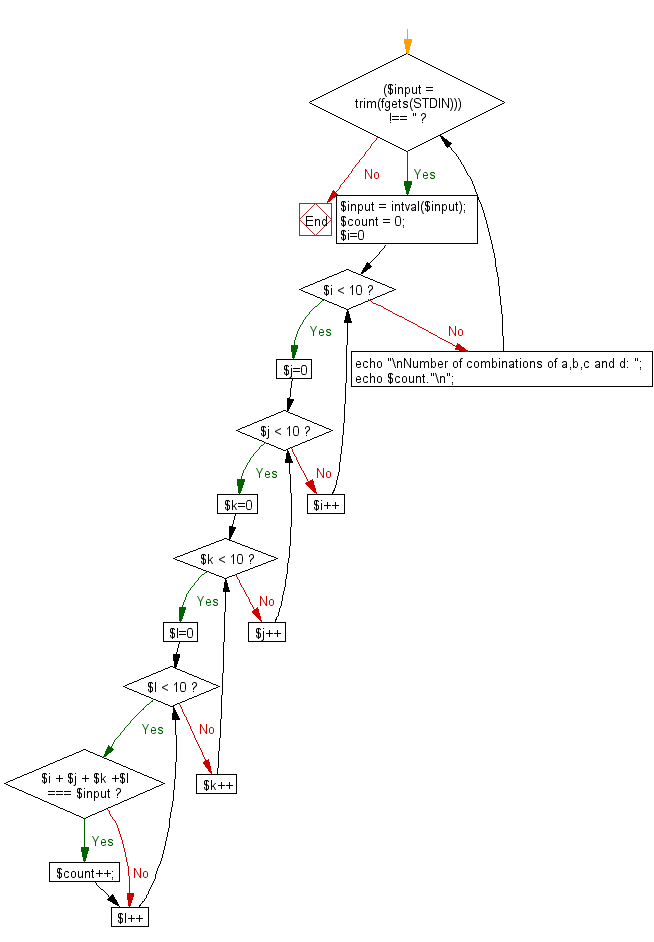﻿ PHP Exercise: Reads an integer n and find the number of combinations - w3resource

# PHP Exercises: Reads an integer n and find the number of combinations

## PHP: Exercise-51 with Solution

Write a PHP program which reads an integer n and find the number of combinations of a,b,c and d (0 ≤ a,b,c,d ≤ 9) where (a + b + c + d) will be equal to n.

Input:
n (1 ≤ n ≤ 50) .

Sample Solution: -

PHP Code:

``````<?php
while ((\$input = trim(fgets(STDIN))) !== '') {
\$input = intval(\$input);
\$count = 0;
for (\$i=0; \$i < 10; \$i++) {
for (\$j=0; \$j < 10; \$j++) {
for (\$k=0; \$k < 10; \$k++) {
for (\$l=0; \$l < 10; \$l++) {
if (\$i + \$j + \$k +\$l === \$input) {
\$count++;
}
}
}
}
}
echo "\nNumber of combinations of a,b,c and d: ";
echo \$count."\n";
}
?>
```
```

Sample Output:

```Number of combinations of a,b,c and d: 56
```

Flowchart:PHP Code Editor:

Have another way to solve this solution? Contribute your code (and comments) through Disqus.

What is the difficulty level of this exercise?

Test your Programming skills with w3resource's quiz.

﻿

## PHP: Tips of the Day

Returns all elements in an array except for the first one

Example:

```<?php
function tips_tail(\$items)
{
return count(\$items) > 1 ? array_slice(\$items, 1) : \$items;
}

print_r(tips_tail([1, 5, 7]));
?>
```

Output:

```Array
(
 => 5
 => 7
)
```